## Summing Amplifier:

As the input impedance of an op-amp is extremely large, more than one input signal can be applied to the inverting amplifier. Such circuit gives the addition of the applied signals at the output. Hence it is called Summer or adder circuit. Depending upon the sign of the output, the Summing Amplifier circuits are classified as inverting summing amplifier and non inverting summing amplifier.

### Inverting Summing Amplifier:

In this circuit, all the input signals to be added are applied to the inverting input terminal, of the op-amp. The circuit with two input signals is shown in the Fig. 2.25.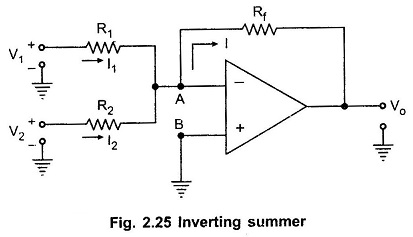As point B is grounded, due to virtual ground concept the node A is also at virtual ground potential.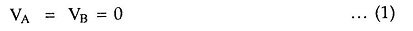Now from input side,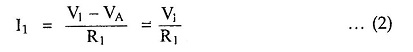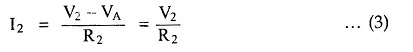Applying at node A and as input op-amp current is zero,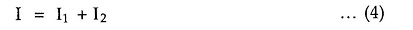From output side,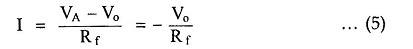Substituting (5), (2) and (3) in (4),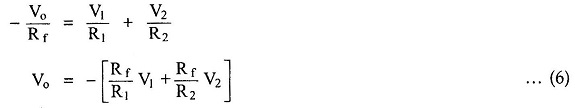If the three resistances are equal, R1 = R2 = Rf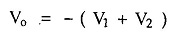By properly selecting Rf, R1 and R2, we can have weighted addition of the input signals like aV+ bV2, as indicated by the equation (6).
Infact in such a way, n input voltages can be added.

Thus the magnitude of the output voltage is the sum of the input voltages and hence circuit is called as summer or adder circuit. Due to the negative sign of the sum at the output it is called inverting summing amplifier. It shows that there is phase inversion.

### Non Inverting Summing Amplifier:

The circuit discussed above is inverting summing op amp, which can be noticed from the negative sign in the equation (6). But a summer that gives non-inverted sum of the input signals is called non inverting summing amplifier. The circuit is shown in the Fig. 2.26.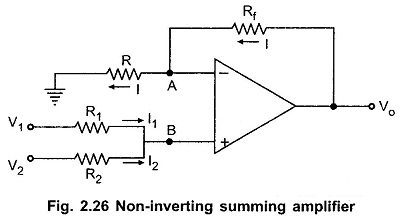Let the voltage of node B is VB. Now the node A is at the same potential as that of B.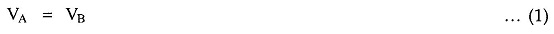From the input side,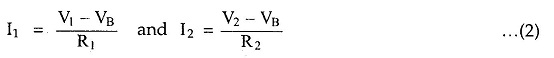But as the input current of op-amp is zero,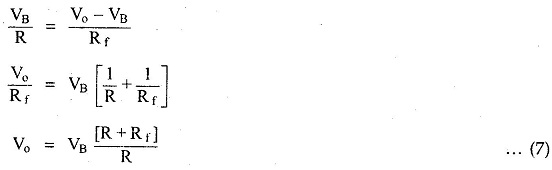Substituting equations (4) in (7) we get,The equation (8) shows that the output is weighted sum of the inputs.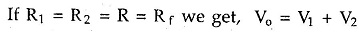As there is no phase difference between input and output, it is called non inverting summing amplifier.

### Average Circuit:

If in the inverting summer amplifier circuit, the values of resistance are selected as,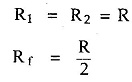then from the equation Vo = V1 + V2) we get,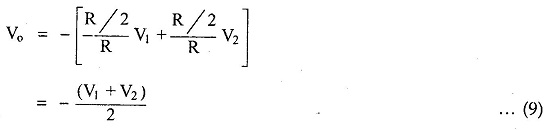Thus the magnitude of the output voltage is the average of the two input voltages. So circuit acts like an averager.
Similarly average of n inputs can be calculated by selecting

Scroll to Top